## QuickLatex

Here is example of a page with LaTeX formulas (how it appears in WordPress editor):

At first, we sample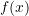in the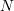(is odd) equidistant points around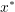: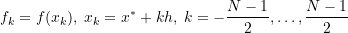where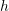is some step.
Then we interpolate points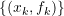by polynomial

(1)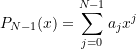Its coefficients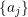are found as a solution of system of linear equations:

(2)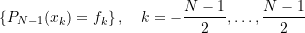Here are references to existing equations: (1), (2).
Here is reference to non-existing equation (??).

Same page processed by QuickLaTeX and published (how visitors see it in a browser):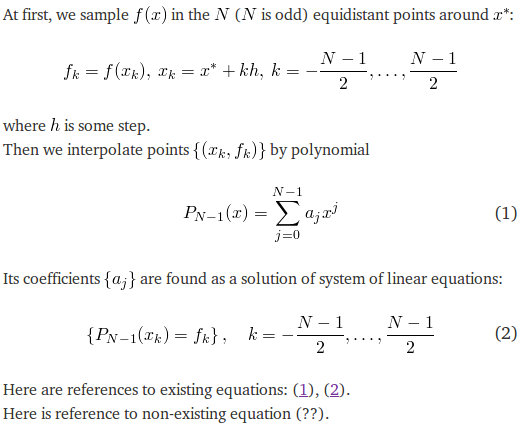For the display environments, equation numbering is automatic, but this may be overridden and the number set explicitly with \tag{..} placed within the display expression.

A number of options may be set for an expression with attribute tags such as size, color, background, align, as arguments of \quicklatex{} placed within the expression: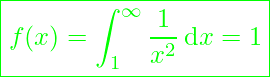renders with green font of 25 pixels height: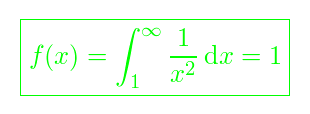Compilation of an expression may be suppressed, showing instead the LaTeX source, by preceding the expression with a !.

For mathematical graphs you may use tikzpicture and pgfplots, e.g. :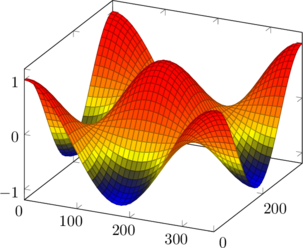compiles to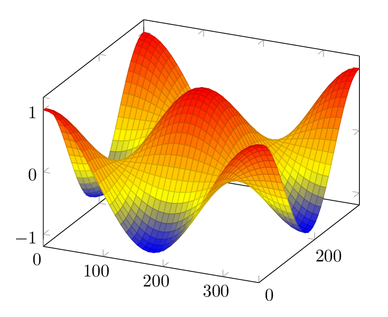Whether or not QuickLaTeX has been activated with , you may always place a LaTeX expression withinshortcodes everywhere on the site. Attribute tags are allowed:.

## KateX

Probando composición tipográfica con LaTeX en eframth.com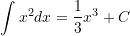(1)alternativas de presentación de ecuaciones.